# Surface Area Of A Cube

Here we will learn about the surface area of a cube, including how to calculate the surface area of a cube and how to find missing values of a cube given its surface area.

There are also surface area of a cube worksheets based on Edexcel, AQA and OCR exam questions, along with further guidance on where to go next if you’re still stuck.

## What is the surface area of a cube?

The surface area of a cube is the sum of the areas of all the faces of a cube. A cube is a three-dimensional solid object that has six congruent square faces. This means they are all the same size.

To find the area of each face we multiply the side lengths together. We then multiply the area of each of the square faces by six.

The formula to calculate the surface area, S, of a cube is

S=6x^{2}

where x represents the side length of the cube.

We can use this formula to find the surface area of any cube.

Surface area is measured in square units, for example mm^{2}, \ cm^{2} or m^{2}.

### What is the surface area of a cube?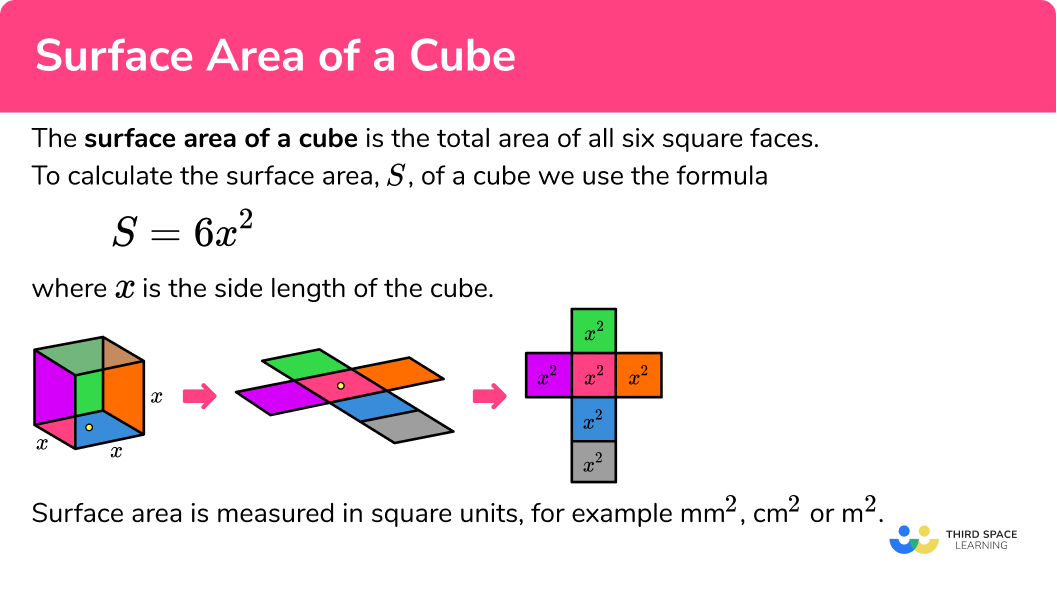## How to calculate the surface area of a cube

In order to calculate the surface area of a cube:

1. Write the formula for the surface area of the cube.
2. Substitute any known value(s) into the formula.
3. Complete the calculation.
4. Write the solution, including the units.

### Explain how to calculate the surface area of a cube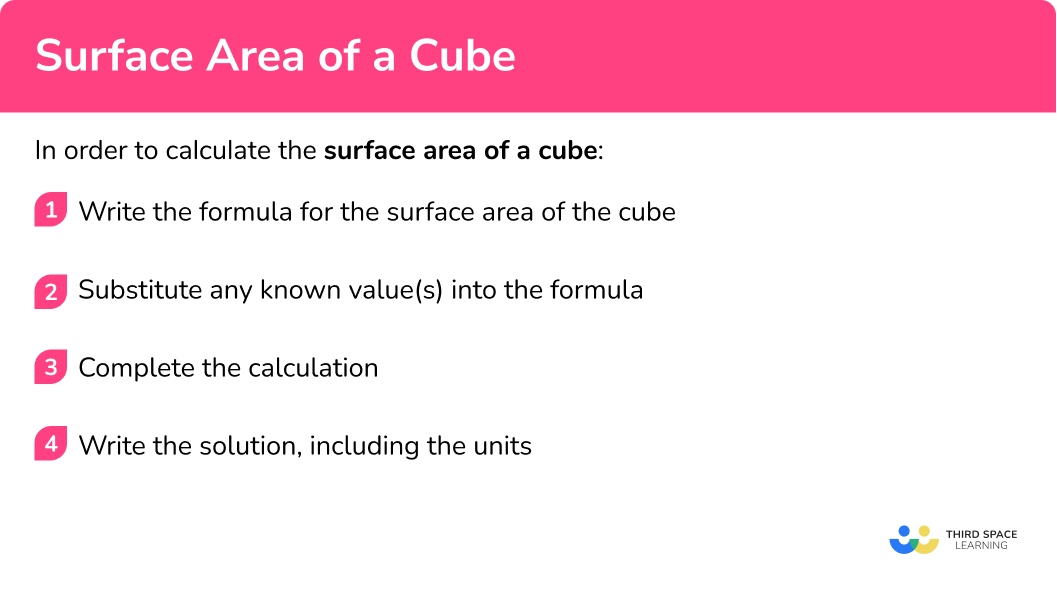## Surface area of a cube examples

### Example 1: integer side lengths

Work out the surface area of the cube below.

1. Write the formula for the surface area of the cube.

S=6x^{2}

2Substitute any known value(s) into the formula.

Here, x=5 and so we have

S=6\times{5}^{2}.

3Complete the calculation.

S=6\times{5}^{2}=6\times{25}=150

4Write the solution, including the units.

As the unit of length is centimetres (cm), the unit of area is square centimetres (cm^{2}).

S=150cm^{2}.

### Example 2: one known edge of the cube

Work out the surface area of the cube.

Write the formula for the surface area of the cube.

Substitute any known value(s) into the formula.

Complete the calculation.

Write the solution, including the units.

### Example 3: worded problem

A cube structure has a side length of 7m. Calculate the total surface area of the structure.

Write the formula for the surface area of the cube.

Substitute any known value(s) into the formula.

Complete the calculation.

Write the solution, including the units.

### Example 4: area of a face given

The area of the face of a cube is 30cm^{2}. Work out the surface area of the cube.

Write the formula for the surface area of the cube.

Substitute any known value(s) into the formula.

Complete the calculation.

Write the solution, including the units.

### Example 5: find the length of a cube given the surface area

The surface area of a cube is 24cm^{2}. Work out the length of the cube.

Write the formula for the surface area of the cube.

Substitute any known value(s) into the formula.

Complete the calculation.

Write the solution, including the units.

### Example 6: find the length of a cube given the surface area (decimal solution)

The surface area of a cube is 483mm^{2}. Work out the length of the side x correct to 2 decimal places.

Write the formula for the surface area of the cube.

Substitute any known value(s) into the formula.

Complete the calculation.

Write the solution, including the units.

### Common misconceptions

• Missing/incorrect units

Surface area is measured in square units (for example, mm^2, \ cm^2, \ m^2 etc.).

• Calculating with different units

You need to make sure all measurements are in the same units before calculating the surface area.

For example, you can’t have some in cm and some in m.

• Calculating volume instead of surface area

Volume and surface area are different quantities. The volume of the cube is the three-dimensional space in a shape and is measured in cubic units.

Surface area is the amount of space covering the outside of a 3D shape. To find surface area, we need to work out the area of one face and multiply it by six.

Surface area of a cuboid is part of our series of lessons to support revision on cuboid. You may find it helpful to start with the main cuboid lesson for a summary of what to expect, or use the step by step guides below for further detail on individual topics. Other lessons in this series include:

### Practice surface area of a cube questions

1. Work out the surface area of the cube.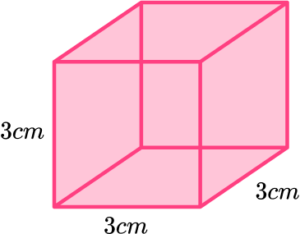27cm^312cm^236cm^254cm^2S=6x^{2} where x=3cm.

S=6\times{3}^{2}=6\times{9}=54cm^{2}.

2. Calculate the surface area of the cube below. Write your answer in square centimetres.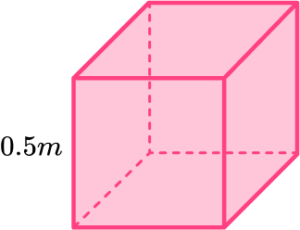0.125cm^315,000cm^26cm^21.5cm^2S=6x^{2} where x=0.5m = 50cm.

S=6\times{50}^{2}=6\times{2500}=15,000cm^{2}.

3. Work out the surface area of the cube. Give your answer in cm^{2}.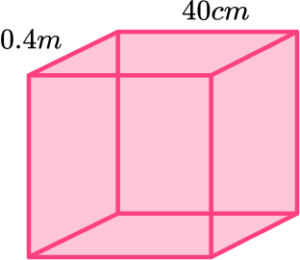96cm^29,600cm^2960cm^29.6cm^2S=6x^{2} where x=40cm=0.4m.

S=6\times{40}^{2}=6\times{1600}=9,600cm^{2}.

4. The surface area of a cube is 150cm^{2}. Find the length of the side of the cube.

135 \ 000cm2.04cm5cm12.5cmS=6x^{2} where S=150cm^{2}.

\begin{aligned} 150&=6\times{x}^{2}\\\\ 25&=x^{2}\\\\ x&=\sqrt{25}\\\\ x&=5 \end{aligned}

5. The surface area of a cube is 6m^{2}. Work out the length of each side x.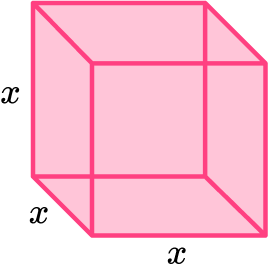2m1m0.3m0.41mS=6x^{2} where S=6m^{2}.

\begin{aligned} 6&=6\times{x}^{2}\\\\ 6\div{6}&=x^{2}\\\\ x^{2}&=1\\\\ x&=\sqrt{1}\\\\ x&=1 \end{aligned}

6. The surface area of a cube is 186m^{2}. Work out the length of each side. Write your answer to the nearest centimetre.

5.57m2.27m15.50m207,576mS=6x^{2} where S=186m^{2}.

\begin{aligned} 186&=6\times{x}^{2}\\\\ 31&=x^{2} \\\\ x&=\sqrt{31}=5.567764362830…=5.57\text{ (2dp)} \end{aligned}

### Surface area of a cube GCSE questions

1. Here is a cube.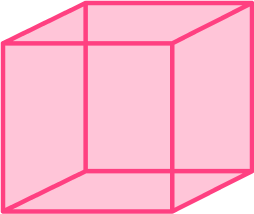The cube has a volume of 216cm^{3}.

Given that V=x^{3}, where x is the side length of the cube, work out the total surface area of the cube.

(4 marks)

x=\sqrt{216} \ (=6cm)

(1)

6 \times 6 \ or \ 36cm^2

(1)

6 \times 6 \times 6 \ or \ 36 \times 6

(1)

216cm^2

(1)

2. The diagram shows a cube of side 3cm.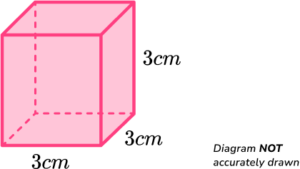Determine the volume : surface area ratio of the cube.

(5 marks)

3 \times 3 \times 6 \ or \ 9 \times 6

(1)

54cm^2

(1)

3 \times 3 \times 3 = 27cm^3

(1)

27:54

(1)

1:2

(1)

3. The total surface area of a cube is 294cm^{2}. Work out the side length of the cube.

(3 marks)

294 \div{6} \ or \ 49

(1)

\sqrt{49}

(1)

7cm

(1)

## Learning checklist

You have now learned how to:

• Calculate the surface area of a cube
• Use the properties of faces, surfaces, edges and vertices of cubes and cuboids to solve problems in 3D

## Still stuck?

Prepare your KS4 students for maths GCSEs success with Third Space Learning. Weekly online one to one GCSE maths revision lessons delivered by expert maths tutors.

Find out more about our GCSE maths tuition programme.